# allcycles

Find all cycles in graph

## Syntax

``cycles = allcycles(G)``
``[cycles,edgecycles] = allcycles(G)``
``[___] = allcycles(G,Name,Value)``

## Description

example

````cycles = allcycles(G)` returns all cycles in the specified graph. The output `cycles` is a cell array where the contents of each cell `cycles{k}` lists nodes that form a cycle.```

example

````[cycles,edgecycles] = allcycles(G)` also returns the edges in each cycle. The output `edgecycles` is a cell array where `edgecycles{k}` gives the edges in the corresponding cycle, `cycles{k}`.```

example

````[___] = allcycles(G,Name,Value)` specifies additional options using one or more name-value arguments. You can use any of the output argument combinations in previous syntaxes. For example, you can specify `MaxNumCycles` and a scalar to limit the number of cycles returned.```

## Examples

collapse all

Create a directed graph with nine nodes. Plot the graph.

```s = [1 2 3 6 5 5 4 6 9 8 8 7]; t = [2 3 6 5 2 4 1 9 8 5 7 4]; G = digraph(s,t); plot(G)```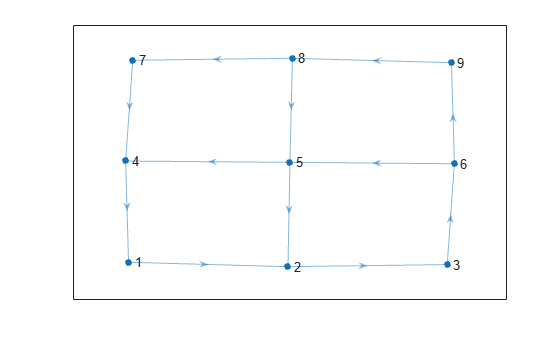Calculate all cycles in the graph.

`cycles = allcycles(G)`
```cycles=5×1 cell array {[ 1 2 3 6 5 4]} {[1 2 3 6 9 8 5 4]} {[1 2 3 6 9 8 7 4]} {[ 2 3 6 5]} {[ 2 3 6 9 8 5]} ```

The second output argument of `allcycles` returns the edges that are contained in each cycle. This is particularly useful for multigraphs, where the edge index is required to uniquely identify the edges in each cycle.

Create a directed multigraph with eight nodes and 18 edges. Specify names for the nodes. Plot the graph with labeled nodes and edges.

```s = [1 1 2 2 3 3 2 2 4 6 8 6 6 7 3 3 5 3]; t = [2 3 1 3 2 1 4 4 6 2 6 7 8 8 5 5 7 7]; names = {'A','B','C','D','E','F','G','H'}; G = digraph(s,t,[],names); p = plot(G,'EdgeLabel',1:numedges(G));```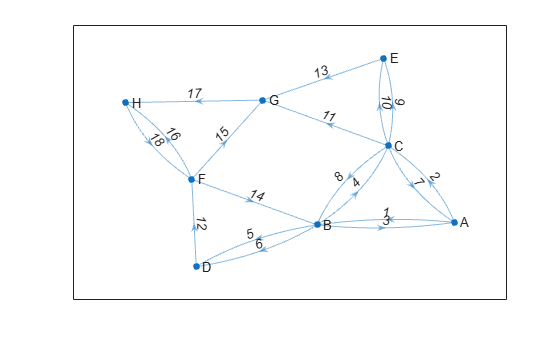Calculate all cycles in the graph. Specify two output arguments to also return the edge indices for edges in each cycle.

`[cycles,edgecycles] = allcycles(G);`

View the nodes and edges in the fifth cycle.

`cycles{5}`
```ans = 1x7 cell {'A'} {'C'} {'E'} {'G'} {'H'} {'F'} {'B'} ```
`edgecycles{5}`
```ans = 1×7 2 9 13 17 18 14 3 ```

Highlight the nodes and edges in the fifth cycle.

`highlight(p,'Edges',edgecycles{5},'EdgeColor','r','LineWidth',1.5,'NodeColor','r','MarkerSize',6)`Use the `'MaxNumCycles'`, `'MaxCycleLength'`, and `'MinCycleLength'` options to limit the number of cycles returned by `allcycles`.

Create an adjacency matrix for a complete graph with 20 nodes. Create an undirected graph from the adjacency matrix, omitting self-loops.

```A = ones(20); G = graph(A,'omitselfloops');```

Since all of the nodes in the graph are connected to all other nodes, there are a large number of cycles in the graph (more than `1.7e17`). Therefore, it is not feasible to calculate all of the cycles since the results will not fit in memory. Instead, calculate the first 10 cycles.

`cycles1 = allcycles(G,'MaxNumCycles',10)`
```cycles1=10×1 cell array {[ 1 2 3]} {[ 1 2 3 4]} {[ 1 2 3 4 5]} {[ 1 2 3 4 5 6]} {[ 1 2 3 4 5 6 7]} {[ 1 2 3 4 5 6 7 8]} {[ 1 2 3 4 5 6 7 8 9]} {[ 1 2 3 4 5 6 7 8 9 10]} {[ 1 2 3 4 5 6 7 8 9 10 11]} {[1 2 3 4 5 6 7 8 9 10 11 12]} ```

Now calculate the first 10 cycles that have a cycle length less than or equal to 3.

`cycles2 = allcycles(G,'MaxNumCycles',10,'MaxCycleLength',3)`
```cycles2=10×1 cell array {[ 1 2 3]} {[ 1 2 4]} {[ 1 2 5]} {[ 1 2 6]} {[ 1 2 7]} {[ 1 2 8]} {[ 1 2 9]} {[1 2 10]} {[1 2 11]} {[1 2 12]} ```

Finally, calculate the first 10 cycles that have a cycle length greater than or equal to 4.

`cycles3 = allcycles(G,'MaxNumCycles',10,'MinCycleLength',4)`
```cycles3=10×1 cell array {[ 1 2 3 4]} {[ 1 2 3 4 5]} {[ 1 2 3 4 5 6]} {[ 1 2 3 4 5 6 7]} {[ 1 2 3 4 5 6 7 8]} {[ 1 2 3 4 5 6 7 8 9]} {[ 1 2 3 4 5 6 7 8 9 10]} {[ 1 2 3 4 5 6 7 8 9 10 11]} {[ 1 2 3 4 5 6 7 8 9 10 11 12]} {[1 2 3 4 5 6 7 8 9 10 11 12 13]} ```

Examine how the outputs of the `cyclebasis` and `allcycles` functions scale with the number of edges in a graph.

Create and plot a square grid graph with three nodes on each side of the square.

```n = 5; A = delsq(numgrid('S',n)); G = graph(A,'omitselfloops'); plot(G)```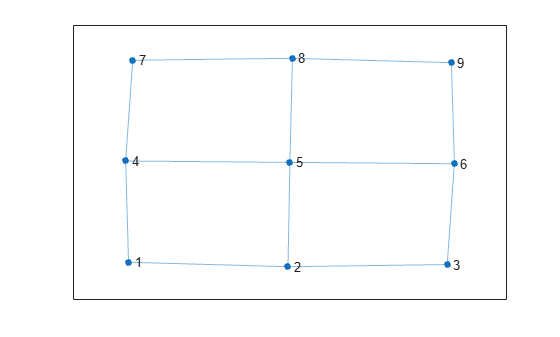Compute all cycles in the graph using `allcycles`. Use the `tiledlayout` function to construct an array of subplots and highlight each cycle in a subplot. The results indicate there are a total of 13 cycles in the graph.

```[cycles,edgecycles] = allcycles(G); tiledlayout flow for k = 1:length(cycles) nexttile highlight(plot(G),cycles{k},'Edges',edgecycles{k},'EdgeColor','r','NodeColor','r') title("Cycle " + k) end```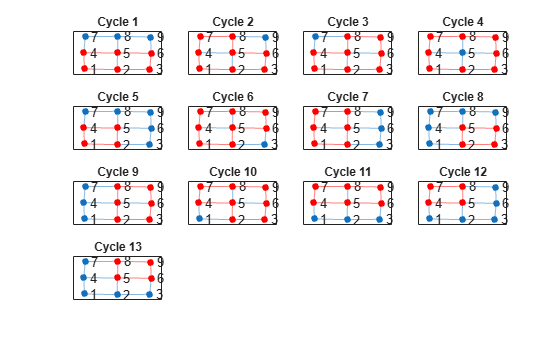Some of these cycles can be seen as combinations of smaller cycles. The `cyclebasis` function returns a subset of the cycles that form a basis for all other cycles in the graph. Use `cyclebasis` to compute the fundamental cycle basis and highlight each fundamental cycle in a subplot. Even though there are 13 cycles in the graph, there are only four fundamental cycles.

```[cycles,edgecycles] = cyclebasis(G); tiledlayout flow for k = 1:length(cycles) nexttile highlight(plot(G),cycles{k},'Edges',edgecycles{k},'EdgeColor','r','NodeColor','r') title("Cycle " + k) end```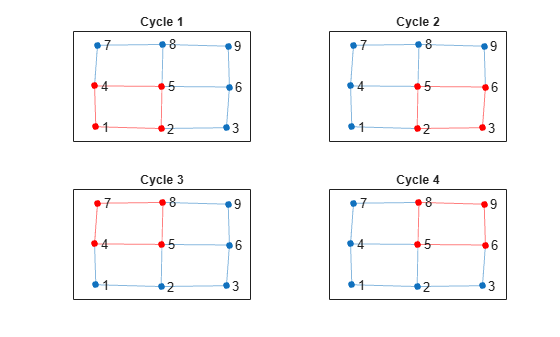Now, increase the number of nodes on each side of the square graph from three to four. This represents a small increase in the size of the graph.

```n = 6; A = delsq(numgrid('S',n)); G = graph(A,'omitselfloops'); figure plot(G)```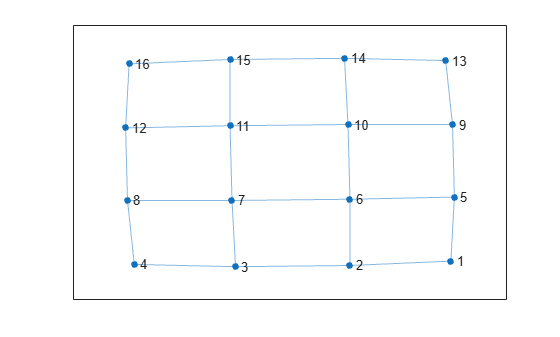Use `allcycles` to compute all of the cycles in the new graph. For this graph there are over 200 cycles, which is too many to plot.

`allcycles(G)`
```ans=213×1 cell array {[ 1 2 3 4 8 7 6 5]} {[ 1 2 3 4 8 7 6 10 9 5]} {[1 2 3 4 8 7 6 10 11 12 16 15 14 13 9 5]} {[ 1 2 3 4 8 7 6 10 11 15 14 13 9 5]} {[ 1 2 3 4 8 7 6 10 14 13 9 5]} {[ 1 2 3 4 8 7 11 10 6 5]} {[ 1 2 3 4 8 7 11 10 9 5]} {[ 1 2 3 4 8 7 11 10 14 13 9 5]} {[ 1 2 3 4 8 7 11 12 16 15 14 10 6 5]} {[ 1 2 3 4 8 7 11 12 16 15 14 10 9 5]} {[ 1 2 3 4 8 7 11 12 16 15 14 13 9 5]} {[1 2 3 4 8 7 11 12 16 15 14 13 9 10 6 5]} {[ 1 2 3 4 8 7 11 15 14 10 6 5]} {[ 1 2 3 4 8 7 11 15 14 10 9 5]} {[ 1 2 3 4 8 7 11 15 14 13 9 5]} {[ 1 2 3 4 8 7 11 15 14 13 9 10 6 5]} ⋮ ```

Despite the large number of cycles in the graph, `cyclebasis` still returns a small number of fundamental cycles. Each of the cycles in the graph can be constructed using only nine fundamental cycles.

```[cycles,edgecycles] = cyclebasis(G); figure tiledlayout flow for k = 1:length(cycles) nexttile highlight(plot(G),cycles{k},'Edges',edgecycles{k},'EdgeColor','r','NodeColor','r') title("Cycle " + k) end```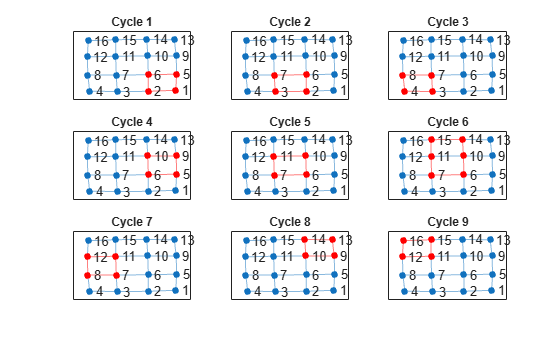The large increase in the number of cycles with only a small change in the size of the graph is typical for some graph structures. The number of cycles returned by `allcycles` can grow exponentially with the number of edges in the graph. However, the number of cycles returned by `cyclebasis` can, at most, grow linearly with the number of edges in the graph.

## Input Arguments

collapse all

Input graph, specified as either a `graph` or `digraph` object. Use `graph` to create an undirected graph or `digraph` to create a directed graph.

Example: `G = graph(1,2)`

Example: `G = digraph([1 2],[2 3])`

### Name-Value Arguments

Specify optional pairs of arguments as `Name1=Value1,...,NameN=ValueN`, where `Name` is the argument name and `Value` is the corresponding value. Name-value arguments must appear after other arguments, but the order of the pairs does not matter.

Before R2021a, use commas to separate each name and value, and enclose `Name` in quotes.

Example: `allcycles(G,'MaxNumCycles',100)` returns only the first 100 cycles in the graph.

Maximum number of cycles, specified as the comma-separated pair consisting of `'MaxNumCycles'` and a nonnegative integer scalar. This option is useful when the number of cycles in a graph grows large enough to hit memory limits. You can specify `MaxNumCycles` to limit the number of cycles returned by `allcycles` so that the results fit within available memory.

Example: `allcycles(G,'MaxNumCycles',100)`

Maximum cycle length, specified as the comma-separated pair consisting of `'MaxCycleLength'` and a positive integer scalar. This option filters the results returned by `allcycles` so that no cycles with length larger than the specified limit are returned. The length of a cycle is measured by the number of edges in it, ignoring edge weights.

To find cycles with a range of lengths, specify both `'MaxCycleLength'` and `'MinCycleLength'`. To find cycles with an exact specified length, specify the same value for both `'MaxCycleLength'` and `'MinCycleLength'`.

Example: `allcycles(G,'MaxCycleLength',4)` returns cycles that have a length less than or equal to 4.

Example: `allcycles(G,'MinCycleLength',3,'MaxCycleLength',5)` returns cycles that have a length of 3, 4, or 5.

Minimum cycle length, specified as the comma-separated pair consisting of `'MinCycleLength'` and a positive integer scalar. This option filters the results returned by `allcycles` so that no cycles with length smaller than the specified limit are returned. The length of a cycle is measured by the number of edges in it, ignoring edge weights.

To find cycles with a range of lengths, specify both `'MaxCycleLength'` and `'MinCycleLength'`. To find cycles with an exact specified length, specify the same value for both `'MaxCycleLength'` and `'MinCycleLength'`.

Example: `allcycles(G,'MinCycleLength',2)` returns cycles that have a length greater than or equal to 2.

Example: `allcycles(G,'MinCycleLength',3,'MaxCycleLength',5)` returns cycles that have a length of 3, 4, or 5.

## Output Arguments

collapse all

Graph cycles, returned as a cell array. Each element `cycles{k}` contains the nodes that belong to one of the cycles in `G`. Each cycle begins with the node that has the smallest node index, and the cycles are returned in lexicographical order. Cycles in undirected graphs are returned only once, following a single direction. If `G` does not contain any cycles, then `cycles` is empty.

The data type of the cells in `cycles` depends on whether the input graph contains node names:

• If graph `G` does not have node names, then each element `cycles{k}` is a numeric vector of node indices.

• If graph `G` has node names, then each element `cycles{k}` is a cell array of character vector node names.

Edges in each cycle, returned as a cell array. Each element `edgecycles{k}` contains the edge indices for edges in the corresponding cycle, `cycles{k}`. If `G` does not contain any cycles, then `edgecycles` is empty.

collapse all

### Graph Cycles

A cycle exists in a graph when there is a nonempty path in which only the first and last nodes are repeated. That is, aside from the first node being repeated at the end of the path to close the cycle, no other nodes are repeated. An example of a cycle is: (Node1 - Node2 - Node3 - Node1). By convention, `allcycles` does not return the last node in the cycle since it is the same as the first.

A cycle cannot traverse the same edge twice. For example, the cycle (Node1 - Node2 - Node1) in an undirected graph only exists if there is more than one edge connecting Node1 and Node2. By this definition, self-loops count as cycles, though they cannot be part of a larger cycle.

## Tips

• The number of cycles in a graph depends heavily on the structure of the graph. For some graph structures, the number of cycles can grow exponentially with the number of nodes. For example, a complete graph with 12 nodes given by ```G = graph(ones(12))``` contains nearly 60 million cycles. Use the `MaxNumCycles`, `MaxCycleLength`, and `MinCycleLength` options to control the output of `allcycles` in these cases.

## Version History

Introduced in R2021a